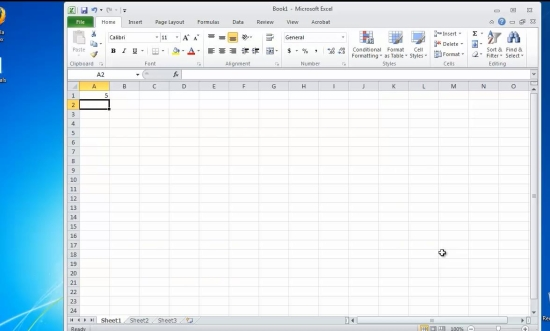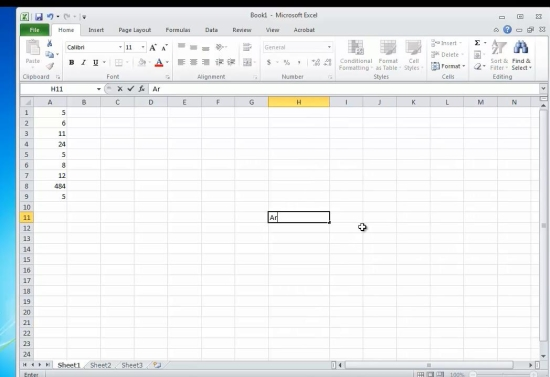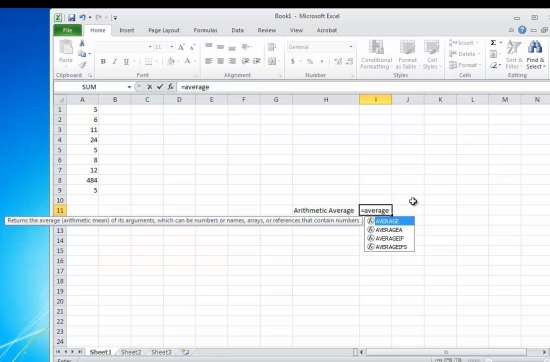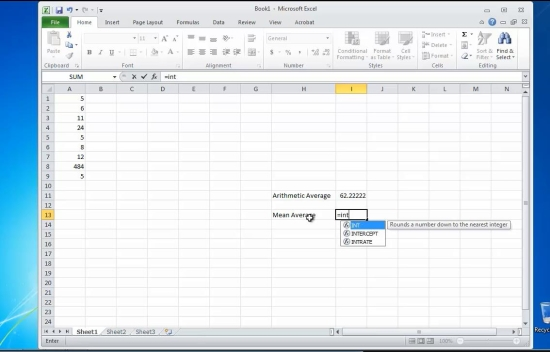## How to Calculate the Average in Excel

In this tutorial you will learn how to access calculate average in Excel

The average is a statistical function that measures central tendency, that is to say the center for a series a numbers. There are three common types of average, depending on the way of calculation.

First is the arithmetical mean, which is calculated by adding all the numbers in a series by their count. The second is the Mean, which is the middle number in a series: there is an equal amount of bigger numbers than it as of smaller numbers. Third averaging technique is simply to collect the most frequent occurring number.

Depending on the type of average you need, there are different ways of generating it.

Let’s start.

Prerequisites: Microsoft Excel.

Step 1. Launch Excel and open create a new document, by selecting File – New.Step 2. Fill in several rows with the values you want to calculate the average for.Step 3. Position your cursor on the cell you want to have the result, and type:

=AVERAGE(Axx:Ayy), where

Axx and Ayy are the start and the end of your series of numbers.

You have obtained the mathematical average for your series of numbers.Step 4. For the second type of average, go to your cell and type the following:

=INT(AVERAGEIF(Axx:Ayy)), where

Axx and Ayy are the start and the end of your series of numbers.

For the third type of average calculation, just use

=MODE(Axx:Ayy), where

Axx and Ayy are the start and the end of your series of numbers.

Press Enter. Excel will show you the result.Result: Congratulations, you have learnt how to calculate the average in Excel.